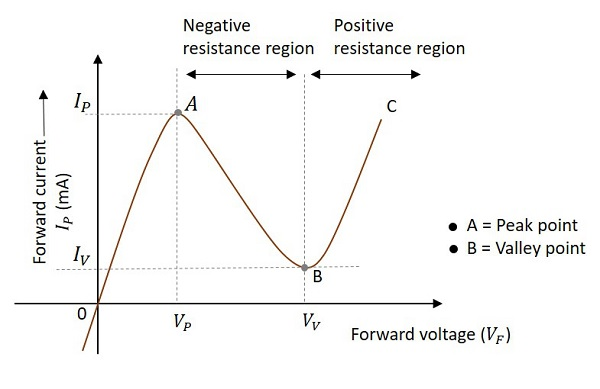# Negative Resistance Oscillators

An oscillator that works on negative resistance property can termed as a Negative resistance oscillator. The term negative resistance refers to a condition where an increase in voltage across two points causes a decrease in current. Some of the non-linear devices exhibit negative resistance property, under certain conditions.

## Negative Resistance Property

Let us observe the behavior when the voltage is applied to a non-linear device that exhibits negative resistance property. To understand this property, let us observe the below graph to find out the variations in voltage and current.As forward voltage increases, the current increases rapidly and it increases until a peak point, called as Peak Current, denoted by IP. The voltage at this point is called as Peak Voltage, denoted by VP. This point is indicated by A in the above graph. The point A is called Peak Point.

If the voltage is further increased beyond VP, then the current starts decreasing. It decreases until a point, called as Valley Current, denoted by IV. The voltage at this point is called as Valley Voltage, denoted by VV. This point is indicated by B in the above graph. The point B is called Valley Point.

Hence the region between point A and point B indicates the Negative resistance region. Once the valley point is reached and if the voltage is further increased, then the current starts increasing. This means the negative resistance region was ended and the device behaves normally as per Ohm’s law. This region is called as Positive Resistance region, which is indicated by point B to point C in the graph.

Few oscillators exhibit negative resistance property during their operation. The uni-junction oscillator is the best example of a non-sinusoidal oscillator (produces sweep waveform as output) that exhibit negative resistance property, while the Tunnel diode oscillator is the best example of a sinusoidal oscillator that exhibit negative resistance property.

In the next chapter of this tutorial, we will discuss more about Tunnel diode oscillators.